# GSEB Solutions Class 12 Maths Chapter 5 Continuity and Differentiability Ex 5.1

Gujarat Board GSEB Textbook Solutions Class 12 Maths Chapter 5 Continuity and Differentiability Ex 5.1 Textbook Questions and Answers.

## Gujarat Board Textbook Solutions Class 12 Maths Chapter 5 Continuity and Differentiability Ex 5.1Question 1.
Prove that the function f(x) = 5x – 3 is continuous at x = 0, at x = – 3 and at x = 5.
Solution:
(i) At x = 0, $$\lim _{x \rightarrow 0}$$f(x) = $$\lim _{x \rightarrow 0}$$ (5x – 3) = – 3
and f(0) = -3.
∴ f is continuous at x = 0.

(ii) At x = – 3, $$\lim _{x \rightarrow -3}$$f(x) = $$\lim _{x \rightarrow -3}$$ (5x – 3) = -18
and f(- 3) = – 18.
∴ f is continuous at x = – 3.

(iii) At x = 5, $$\lim _{x \rightarrow 5}$$ f(x) = $$\lim _{x \rightarrow 5}$$ (5x – 3) = 22
and f(5) = 22
∴ f is continuous at x = 5.

Use this handy free online Implicit Differentiation Calculator tool to get the accurate output of an equation in a fraction of seconds.Question 2.
Examine the continuity of the function f(x) = 2x² – 1 at x = 3.
Solution:
$$\lim _{x \rightarrow 3}$$ f(x) = $$\lim _{x \rightarrow 3}$$ (2x² – 1) = 17
and f(3) = 17.
∴ f is continuous at x = 3.

Question 3.
Examine the following functions for continuity:
(a) f(x) = x – 5
(b) f(x) = $$\frac { 1 }{ x-5 }$$
(c) f(x) = $$\frac{x^{2}-25}{x+5}$$
(d) f(x) = |x-5|
Solution:
(a) f(x) = x – 5
x – 5 is a polynomial.
Therefore, it is continuous at each x ∈ R.

(b) f(x) = $$\frac { 1 }{ x-5 }$$
At x = 5, f(x) is not defined.
∴ f is not continuous at x = 5.
When x ≠ 5, $$\lim _{x \rightarrow c}$$ = $$\frac { 1 }{ c-5 }$$
Also, f(c) = $$\frac { 1 }{ c-5 }$$
∴ f is continuous at x ∈ R – {5}.

(c) f(x) = $$\frac{x^{2}-25}{x+5}$$
At x = – 5, function/is not defined.
∴ f is discontinuous at x = – 5.
At x = c ≠ – 5,
$$\lim _{x \rightarrow c}$$ f(x) = $$\lim _{x \rightarrow c}$$ $$\frac{x^{2}-25}{x+5}$$ = c – 5
and f(c) = c – 5.
∴ f is continuous for all x ∈ R – {- 5).

(d) f(x) = |x – 5|
At x = 5, f(5) = |5 – 5| = 0
$$\lim _{x \rightarrow c}$$ |x – 5| = 0
∴ f is continuous at x = 5.
At x = c > 5,
$$\lim _{x \rightarrow c}$$ |x – 5| = c – 5 [c > 5]
Also, f(c) = c – 5.
∴ f is continuous at x = c > 5.
Similarly, at x = c < 5,
$$\lim _{x \rightarrow c}$$ |x – 5| = 5 – c and f(c) = 5 – c.
∴ f is continuous at x = c < 5.
Thus, f is continuous for all x ∈ R.Question 4.
Prove that the function f(x) = xn is continuous at x = n, when n is a positive integer.
Solution:
f(x) = xn is a polynomial which is continuous for all x ∈ R.
Hence, f is continuous at x = n, n ∈ N.

Question 5.
Is the function f defined by
f(x) = $$\left\{\begin{array}{l} x, \text { if } x \leq 1 \\ 5, \text { if } x>1 \end{array}\right.$$
continuous at x = 0?, At x = 1?, At x = 2?
Solution:
(i) At x = 0,
$$\lim _{x \rightarrow 0^{-}}$$ f(x) = $$\lim _{x \rightarrow 0^{-}}$$ x = 0.
$$\lim _{x \rightarrow 0^{+}}$$ f(x) = $$\lim _{x \rightarrow 0^{+}}$$ x = 0.
Also, f(0) = 0.
∴ f is continuous at x = 0.

(ii) At x =1,
$$\lim _{x \rightarrow 1^{-}}$$ f(x) = $$\lim _{x \rightarrow 1^{-}}$$ (x) = 1.
$$\lim _{x \rightarrow 1^{+}}$$ f(x) = $$\lim _{x \rightarrow 1^{+}}$$ (x) = 5.
$$\lim _{x \rightarrow 1^{-}}$$ f(x) ≠ $$\lim _{x \rightarrow 1^{+}}$$ (x)
∴ f is discontinuous at x = 1.

(iii) At x = 2,
$$\lim _{x \rightarrow 2}$$ f(x) = 5 and f(2) = 5.
∴ f is continuous at x = 2.Question 6.
f(x) = $$\left\{\begin{array}{l} 2 x+3, x \leq 2 \\ 2 x-3, x>2 \end{array}\right.$$
Solution:
f(x) = $$\left\{\begin{array}{l} 2 x+3, x \leq 2 \\ 2 x-3, x>2 \end{array}\right.$$
At x – 2, L.H.L. = $$\lim _{x \rightarrow 2^{-}}$$ (2x + 3) = 7
f(2) = 2 x 2 + 3 = 7
R.H.L. = $$\lim _{x \rightarrow 2^{+}}$$ (2x – 3) = 2 x 2 – 3 = 1
⇒ L.H.L. ≠ R.H.L.
∴ f is discontinuous at x = 2.
At x = c < 2, $$\lim _{x \rightarrow c}$$ (2x + 3) = 2c + 3 = f(c).
f is continuous at x = c < 2. At x = c > 2, $$\lim _{x \rightarrow c}$$ (2x – 3) = 2c – 3 = f(c).
∴ f is continuous at x = c > 2.
⇒ Point of discontinuity is x = 2.

Question 7.
f(x) = $$\left\{\begin{array}{l} |x|+3, \text { if } x \leq-3 \\ -2 x, \quad \text { if }-3<x<3 \\ 6 x+2, \text { if } x>3 \end{array}\right.$$
Solution:
f(x) = $$\left\{\begin{array}{l} |x|+3, \text { if } x \leq-3 \\ -2 x, \quad \text { if }-3<x<3 \\ 6 x+2, \text { if } x>3 \end{array}\right.$$
At x = – 3, L.H.L. = $$\lim _{x \rightarrow 3^{-}}$$ (|x|+3)
= $$\lim _{x \rightarrow -3^{-}}$$ (-x + 3) = 3 + 3 = 6.
f(- 3) = |- 3| + 3 = 6.
R.H.L. = $$\lim _{x \rightarrow -3^{+}}$$ f(x) = $$\lim _{x \rightarrow -3^{+}}$$ (- 2x) = 6.
L.H.L. = R.H.L. = f(- 3).
⇒ f is continuous at x = – 3.
At x = 3, L.H.L. = $$\lim _{x \rightarrow 3^{-}}$$ f(x) = $$\lim _{x \rightarrow 3^{-}}$$ (- 2x) = – 6
R.H.L. = $$\lim _{x \rightarrow 3^{+}}$$ f(x) = $$\lim _{x \rightarrow 3^{+}}$$ (6x + 2) = 20
f(3) is not defined.
∴ L.H.L. ≠ R.H.L. ≠ f(3)
∴ f is discontinuous at x = 3.
At x = c < – 3,
$$\lim _{x \rightarrow -c}$$ (|x| + 3) = – c + 3 = f(c)
⇒ $$\lim _{x \rightarrow -c}$$ f(x) = f(c)
⇒ f is continuous at x = c < – 3.
At x = c, when – 3 < x < 3,
$$\lim _{x \rightarrow 1^{-}}$$ (- 2x) =-2c =f(c) ⇒ $$\lim _{x \rightarrow 1^{-}}$$ f(x) = f(c).
∴ f is continuous at x = c, where -3 < c < 3.
At x = c, $$\lim _{x \rightarrow c}$$(6x + 2) = 6c + 2 = f(c).
⇒ $$\lim _{x \rightarrow c}$$f(x) = f(c)
⇒ f is continuous at x = c > 3.Question 8.
f(x) = $$\left\{\begin{array}{ll} \frac{|x|}{x}, & \text { if } x \neq 0 \\ 0, & \text { if } x=0 \end{array}\right.$$
Solution: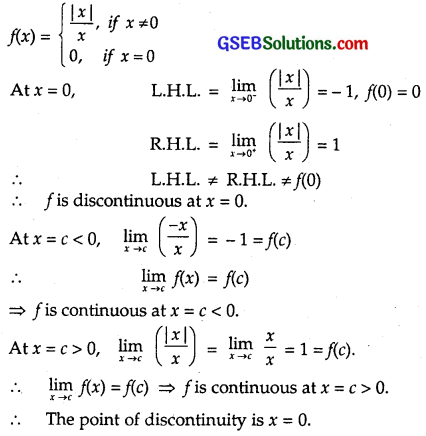Question 9.
f(x) = $$\left\{\begin{array}{ll} \frac{x}{|x|}, & \text { if } x<0 \\ -1, & \text { if } x \geq 0 \end{array}\right.$$
Solution: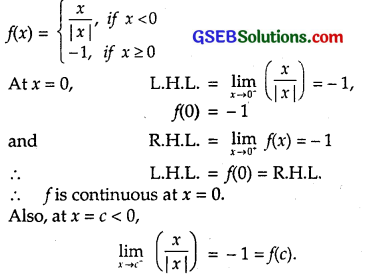∴ $$\lim _{x \rightarrow c}$$f(x) = f(c) ⇒ f is continuous at x = c < 0. At x = c > 0, $$\lim _{x \rightarrow c}$$ f(x) = – 1
Also, f(c) = – 1.
∴ $$\lim _{x \rightarrow c}$$ f(x) = f(c) ⇒ f is continuous at x = c > 0.
There is no point of discontinuity for this function in its domain.Question 10.
f(x) = $$\left\{\begin{array}{ll} x+1, & \text { if } x \geq 1 \\ x^{2}+1, & \text { if } x<1 \end{array}\right.$$
Solution:
f(x) = $$\left\{\begin{array}{ll} x+1, & \text { if } x \geq 1 \\ x^{2}+1, & \text { if } x<1 \end{array}\right.$$
At x = 1, L.H.L. = $$\lim _{x \rightarrow 1^{-}}$$ f(x) = $$\lim _{x \rightarrow 1^{-}}$$ (x² + 1) = 2,
R.H.L. = $$\lim _{x \rightarrow 1^{+}}$$ f(x) = $$\lim _{x \rightarrow 1^{+}}$$ (x + 1) = 2
and f(1) = 1 + 1 = 2.
⇒ f is continuous at x = 1.
At x = c > 1, $$\lim _{x \rightarrow c}$$ f(x) = $$\lim _{x \rightarrow c}$$ (x + 1) = c + 1 = f(c).
⇒ f is continuous at x = c > 1.
At x = c < 1, $$\lim _{x \rightarrow c}$$ f(x) = $$\lim _{x \rightarrow c}$$ (x² + 1) = c² + 1 = f(c).
⇒ f is continuous at x = c < 1.
There is no point of discontinuity at any noint x ∈R.

Question 11.
f(x) = $$\left\{\begin{array}{l} x^{3}-3, \text { if } x \leq 2 \\ x^{2}+1, \text { if } x>2 \end{array}\right.$$
Solution:
$$\left\{\begin{array}{l} x^{3}-3, \text { if } x \leq 2 \\ x^{2}+1, \text { if } x>2 \end{array}\right.$$
At x = 2,
L.H.L. = $$\lim _{x \rightarrow 2^{-}}$$ (x³ – 3) = 8 – 3 = 5,
R.H.L. = $$\lim _{x \rightarrow 2^{+}}$$ (x² + 1) = 4 + 1 = 5
and f(2) = 2³ – 3 = 8 – 3 = 5.
⇒ f is continuous at x = 2.
At x = c < 2, $$\lim _{x \rightarrow c}$$ (x³ – 3) = c³ – 3 = f(c).
⇒ f is continuous at x < 2.
At x = c > 2, $$\lim _{x \rightarrow c}$$ (x² + 1) = c² + 1 = f(c).
⇒ f is continuous at x > 2.
Hence, f is continuous for all x ∈ R.
∴ There is no point of discontinuity.

Question 12.
f(x) = $$\left\{\begin{array}{l} x^{10}-1, \text { if } x \leq 1 \\ x^{2}, \quad \text { if } x>1 \end{array}\right.$$
Solution: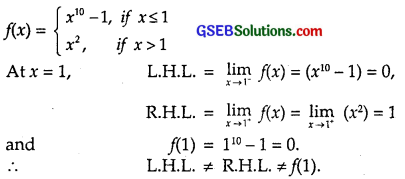⇒ f is continuous at x = 1.
At x = c &lt; 1, $$\lim _{x \rightarrow c}$$ (x<sup>10</sup> – 1) = c<sup>10</sup> – 1 = f(c).
⇒ f is continuous at x &lt; 1.
At x = c &gt; 1, $$\lim _{x \rightarrow c}$$ (x²) = c² = f(c).
⇒ f is continuous at x &gt; 1.
Hence, f is continuous at all points x ∈ R – 
∴ Point of discontinuity is x = 1.Question 13.
Is the function defined by
f(x) = $$\left\{\begin{array}{l} x+5, \text { if } x \leq 1 \\ x-5, \text { if } x>1 \end{array}\right.$$
a continuous function?
Solution:
At x = 1,
L.H.L. = $$\lim _{x \rightarrow 1^{-}}$$ f(x) = $$\lim _{x \rightarrow 1^{-}}$$ (x + 5) = 6,
R.H.L. = $$\lim _{x \rightarrow 1^{+}}$$ f(x) = $$\lim _{x \rightarrow 1^{+}}$$ (x – 5) = – 4,
and
f(1) = 1 + 5 = 6
f(1) = L.H.L ≠ R.H.L
⇒ f is continuous at x = 1.
At x = c < 1, $$\lim _{x \rightarrow c}$$ (x + 5) = c + 5 = f(c).
⇒ f is continuous at x < 1.
At x = c > 1, $$\lim _{x \rightarrow c}$$ (x – 5) = c – 5 = f(c).
⇒ f is continuous at x > 1.
⇒ f is continuous at all points x ∈ R, except x = 1.

Question 14.
f(x) = $$\left\{\begin{array}{l} 3, \text { if } 0 \leq x \leq 1 \\ 4, \text { if } 1<x<3 \\ 5, \text { if } 3 \leq x \leq 10 \end{array}\right.$$
Solution:
f(x) = $$\left\{\begin{array}{l} 3, \text { if } 0 \leq x \leq 1 \\ 4, \text { if } 1<x<3 \\ 5, \text { if } 3 \leq x \leq 10 \end{array}\right.$$
In the interval 0 ≤ x < 1, f(x) = 3.
f is continuous in this interval.
At x = 1,
L.H.L. = $$\lim _{x \rightarrow 1^{-}}$$ f(x) = 3
R.H.L. = $$\lim _{x \rightarrow 1^{+}}$$ f(x) = 4
⇒ f is discontinous at x = 1 because L.H.L. ≠ RH.L
In the interval 1 < x < 3, f(x) = 4.
∴ f is continuous in this interval.
At x = 3,
L.H.L. = $$\lim _{x \rightarrow 3^{-}}$$ f(x) = 4
R.H.L. = $$\lim _{x \rightarrow 3^{+}}$$ f(x) = 5
⇒ f is discontinous at x = 1 because L.H.L. ≠ RH.L
In the interval 3 ≤ x ≤ 10, f(x) = 5.
∴ f is continuous in this interval except for x = 3
⇒ f is not continous at x = 1 and x = 3.Question 15.
f(x) = $$\left\{\begin{array}{l} 2 x, \text { if } x<0 \\ 0, \text { if } 0 \leq x \leq 1 \\ 4 x, \text { if } x>1 \end{array}\right.$$
Solution:
At x = 0,
L.H.L. = $$\lim _{x \rightarrow 0^{-}}$$ (2x) = 0
R.H.L. = $$\lim _{x \rightarrow 0^{+}}$$ (0) = 0
and f(0) = 0.
⇒ f is continous at x = 0.
At x = 1,
L.H.L. = $$\lim _{x \rightarrow 1^{+}}$$ (0) = 0
R.H.L. = $$\lim _{x \rightarrow 1^{+}}$$ (4x) = 4
and f(1) = 0.
∴ f(1) = L.H.L. ≠ R.H.L.
∴ f is continuous at x = 1.
When x < 0, f(x) = 2x, being a polynomial, is continuous at all points x < 0. When x > 1, f(x) = 4x, being a polynomial, is continuous at all points x > 1.
When 0 ≤ x ≤ 1, f(x) = 0 is a continuous function, except for the point x = 1.
∴ The point of discontinuity is x =1.

Question 16.
f(x) = $$\left\{\begin{array}{ll} -2, & \text { if } x<-1 \\ 2 x, & \text { if }-1<x<1 \\ 2, & \text { if } x>1 \end{array}\right.$$
Solution:
At x = – 1,
L.H.L. = $$\lim _{x \rightarrow 1^{-}}$$ f(x) = -2,
f(-1) = – 2
and R.H.L. = $$\lim _{x \rightarrow 1^{+}}$$ f(x) = – 2.
⇒ f is continous at x = – 1.
At x = 1,
L.H.L. = $$\lim _{x \rightarrow 1^{-}}$$ f(x) = 2
f(1) = 2
R.H.L. = $$\lim _{x \rightarrow 1^{+}}$$ f(x) = 2
∴ f is continuous at x = 1.
Hence, f is continuous function.Question 17.
Find the values of a and b so that the function defined by
f(x) = $$\left\{\begin{array}{ll} a x+1, & \text { if } x \leq 3 \\ b x+3, & \text { if } x>3 \end{array}\right.$$
is continuous at x = 3.
Solution: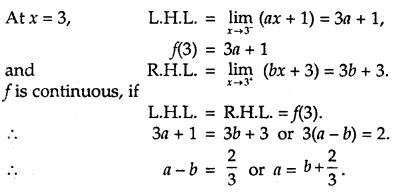For any arbitary value of b, we can find the value of a corresponding to the value of b. Thus, there are infinitely many values of a and b.

Question 18.
For what value of λ, is the function
f(x) = $$\left\{\begin{array}{l} \lambda\left(x^{2}-2 x\right), \text { if } x \leq 0 \\ 4 x+1, \quad \text { if } x>0 \end{array}\right.$$
continuous at x = 0? What about continuity at x = 1?
Solution: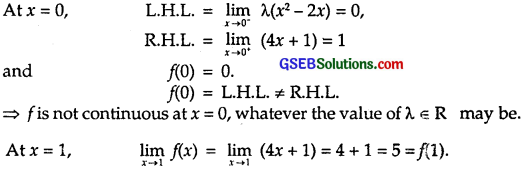So, f is continuous at x = 1.
⇒ f is not continuous at x = 0 for any value of λ, but f is continuous at x = 1 for all values of λ.Question 19.
Show that function defined by g(x) = x – [x] is discontinuous at all integral points. Here, [x] denotes the greatest integer less than or equal to x.
Solution:
Let c be an integer.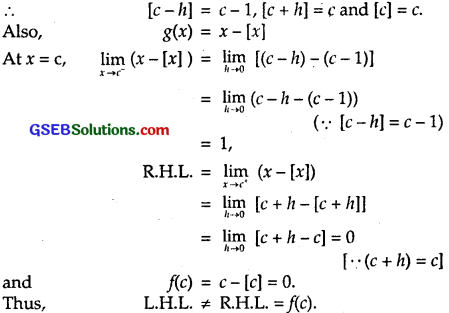⇒ f is not continuous at all integral points.

Note:
To find :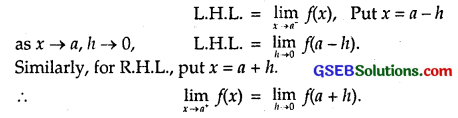Question 20.
Is the function defined by x² – sin x + 5 continuous at x = π?
Solution:
Let f(x) = x² – sin x + 5.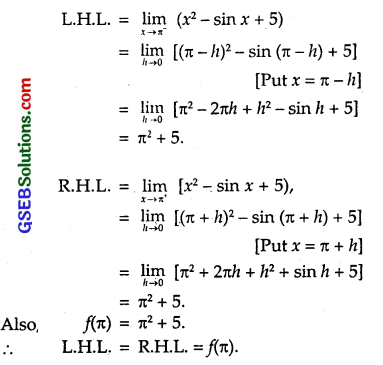Hence, f is continuous at x = π.

Alternatively:
g(x) = x² + 5 is a polynomial and h(x) = sin x
∴ g is continuous for all x ∈ R.
h(x) = sin x, which is continuous for all x ∈ R
∴ f = g – h is also continuous for x ∈ R.

Question 21.
Discuss the continuity of the following functions:
(a) f(x) = sin x + cos x
(b) f(x) = sin x – cos x
(c) f(x) = sin x.cos x
Solution: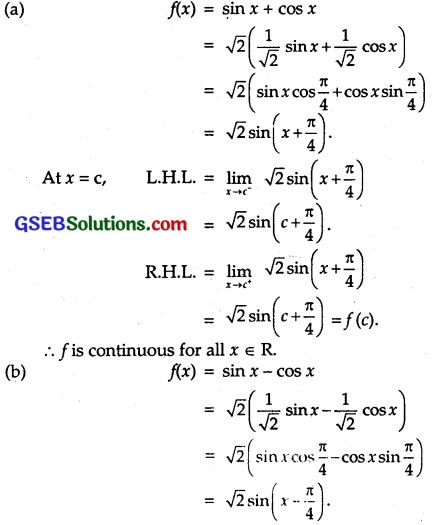As above, f is continuous for all x ∈ R.

(c)
f(x) = sin x cos x = $$\frac { 1 }{ 2 }$$(2 sin x cos x)
= $$\frac { 1 }{ 2 }$$ sin 2x.
Again, f is continuous for all x ∈ R.Question 22.
Discuss the continuity of the cosine, cosecant, secant and cotangent functions.
Solution:
Let f(x) = cos x.
At x = c, c ∈ R,
$$\lim _{x \rightarrow c}$$ cos x = cos c = f(c).
∴ f is continuous for all values of x ∈ R.

(b) Let f(x) = sec x.
sec x is not defined at x = (2n + 1) $$\frac { π }{ 2 }$$, n ∈Z.
Also, at x = $$\frac { π }{ 2 }$$,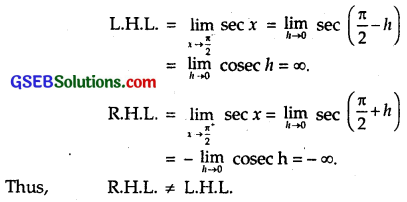∴ f is not continuous at x = $$\frac { π }{ 2 }$$ or at x = (2n + 1) $$\frac { π }{ 2 }$$.
At x = c ≠ (2n + 1)$$\frac { π }{ 2 }$$,
$$\lim _{x \rightarrow c}$$ sec x = sec c = f(c).
Hence, f is continuous at x ∈ R except at x = (2n + 1) $$\frac { π }{ 2 }$$, where n ∈ Z.

(c) f(x) = cosec x
f is not defined at x = nπ.
⇒ f is not continuous at x = nπ.

(d) f(x) = cot x
f is not defined at x = nπ.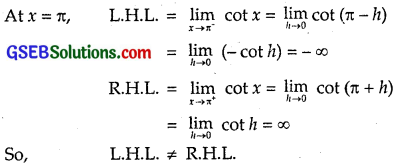Thus, f is not continuous at x = π or at x = nπ.
At x = c ≠ nπ,
$$\lim _{x \rightarrow c}$$ cot x = cot c = f(c).
f is continuous at all points x ∈ R except x = nπ, where n ∈ Z.

Question 23.
Find all the points of discontinuity of f, where
f(x) = $$\left\{\begin{array}{l} \frac{\sin x}{x}, \text { if } x<0 \\ x+1, \text { if } x \geq 0 \end{array}\right.$$
Solution: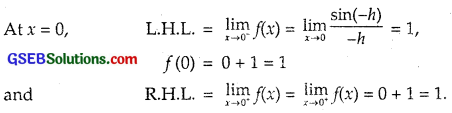∴ f is continuous at x = 0.
When x < 0, sin x and x both are continuous.
∴ $$\frac { sin x }{ x }$$ is also continuous.
When x > 0, f(x) = x + 1 is a polynomial.
∴ f is continuous.
⇒ f is not discontinuous at any point.

Question 24.
Determine if f defined by f(x) = $$\left\{\begin{array}{l} x^{2} \sin \frac{1}{x}, \text { if } x \neq 0 \\ 0, \quad \text { if } x=0 \end{array}\right.$$ is a continuous function?
Solution: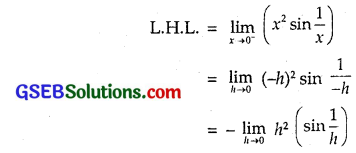sin $$\frac { 1}{ h }$$ lies between -1 and 1, a finite quantity.
∴ h² sin $$\frac { 1}{ h }$$ → 0 as h → 0.
∴ L.H.L. = 0
Similarly, $$\lim _{x \rightarrow 0^{+}}$$(x² sin $$\frac { 1}{ h }$$) = 0
Also, f(0) = 0 [Gievn]
∴ L.H.L = R.H.L = f(c)
∴ f is continuous for all x ∈ R.Question 25.
Examine the continuity of f, where f is defined by f(x) = $$\left\{\begin{array}{r} \sin x-\cos x, \text { if } x \neq 0 \\ -1, \quad \text { if } x=0 \end{array}\right.$$
Solution: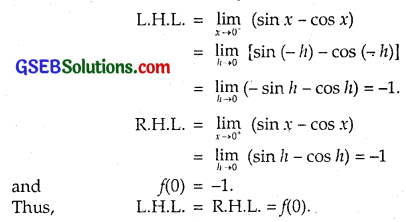∴ f is continuous at x = 0.

Alternatively :
sin x and cos x both are continuous for all x ∈ R.
∴ sin x – cos x is continuous for all x ∈ R.
⇒ f is continuous for all x ∈ R for all x ∈ R.

Question 26.
f(x) = $$\left\{\begin{array}{r} \frac{k \cos x}{\pi-2 x}, \text { if } x \neq \frac{\pi}{2} \\ 3, \text { if } x=\frac{\pi}{2} \end{array}\right. \text { at } x=\frac{\pi}{2}$$
Solution: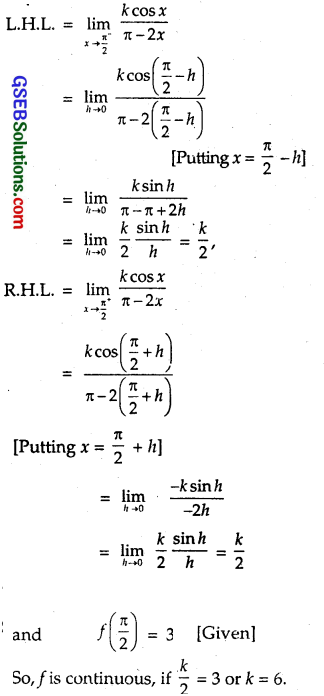Question 27.
The function is defined by
f(x) = $$\left\{\begin{array}{l} k x^{2}, \text { if } x \leq 2 \\ 3, \text { if } x>2 \end{array}\right. \text { at } x=2$$
Solution: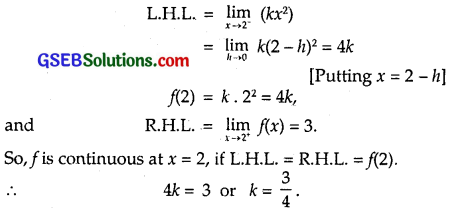Question 28.
The function is defined by
f(x) = $$\left\{\begin{array}{ll} k x+1, & \text { if } x \leq \pi \\ \cos x, & \text { if } x>\pi \end{array}\right. \text { at } x=\pi$$
Solution: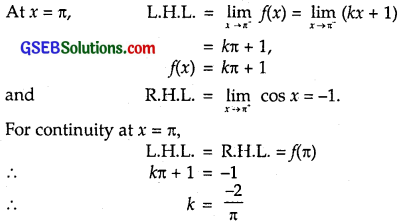Question 29.
The function is defined by
f(x) = $$\left\{\begin{array}{l} k x+1, \text { if } x \leq 5 \\ 3 x-5, \text { if } x>5 \end{array}\right. \text { at } x=5$$
Solution:
L.H.L. = $$\lim _{x \rightarrow 5^{-}}$$ (kx + 1) = 5k +1,
f(5) = k . 5 + 1 – 5k + 1
and
R.H.L. = $$\lim _{x \rightarrow 5^{+}}$$ (3x – 5) = 10.
f is continuous, if L.H.L. = R.H.L. = f(5).
∴ 5k + 1 = 10
∴ k = $$\frac { 9 }{ 5 }$$.Question 30.
Find the values of a and b such that the function defined by
f(x) = $$\left\{\begin{array}{cl} 5, & \text { if } x \leq 2 \\ a x+b, & \text { if } 2<x<10 \\ 21, & \text { if } x \geq 10 \end{array}\right.$$
is a continous function.
Solution:
At x = 2, L.H.L. = $$\lim _{x \rightarrow 2^{-}}$$ (5) = 5,
f(2) = 5
and R.H.L. = $$\lim _{x \rightarrow 2^{+}}$$ (ax + b) = 2a + b.
f is continuous at x = 2, if 2a + b = 5 … (1)
At x = 10, L.H.L. = $$\lim _{x \rightarrow 10^{-}}$$ f(x) = $$\lim _{x \rightarrow 10^{-}}$$ (ax + b)
= 10a + b
and R.H.L. = $$\lim _{x \rightarrow 10^{+}}$$ f(x) = $$\lim _{x \rightarrow 10^{+}}$$ (21) = 21.
Also, f(10) = 21.
∴ f is continuous at x = 10, if 10a + b = 21. … (2)
Subtracting (1) from (2),
8a = 21 – 5 = 16. ∴ a = 2.
From (1), 2 x 2 + b – 5. ∴ b = 1.
Hence, a = 2 and b = 1.

Question 31.
Show that the function defined by f(x) = cos x² is a continuous function.
Solution:
Now, f(x) = cos x².
Let g(x) = cos x and h(x) = x².
∴ (goh) (x) = g(h(x)) = cos x².
Now, g and h both are continuous for all x ∈ R.
∴ f(x) = (goh)(x) = cos x² is also continuous at all x ∈ R.Question 32.
Show that the function defined by f(x) = | cos x | is a continuous function.
Solution:
Let g(x) = | x | and f(x) – cos x.
∴ f(x) = (goh)(x) = g(h(x))
= g(cos x) = | cos x|.
Now, g{x) = | x | and h(x) = cos x
both are continuous for all values of x ∈ R.
∴ (goh)(x) is also continuous.
Hence, f(x) = (goh)(x)
= | cos x | is continuous for all values of x ∈ R.

Question 33.
Examine if sin | x | is a continuous function.
Solution:
Let g(x) = sin x and h(x) = | x |.
∴ (goh)(x) = g(h(x)) = g(| X |) = sin | x | = f(x).
Now, g(x) = sin x and h(x) = | x |
both are continuous for all x ∈ R.
∴ f(x) = (goh)(x)
= sin | x | is continuous at all x ∈ R.Question 34.
Find all the points of discontinuity of f defined by f(x) = |x| – |x+1|.
Solution: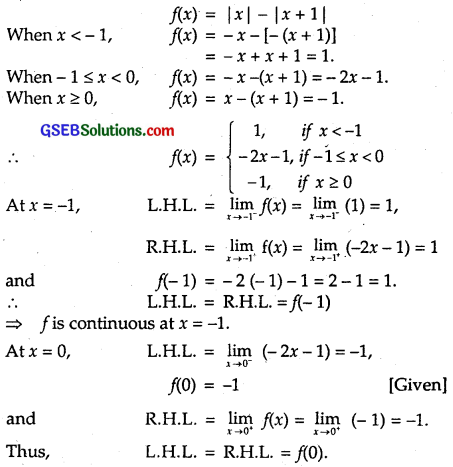∴ f is continous at x = 0
⇒ There is no point of discontinuity.
Hence, f is continuous for all x ∈ R.